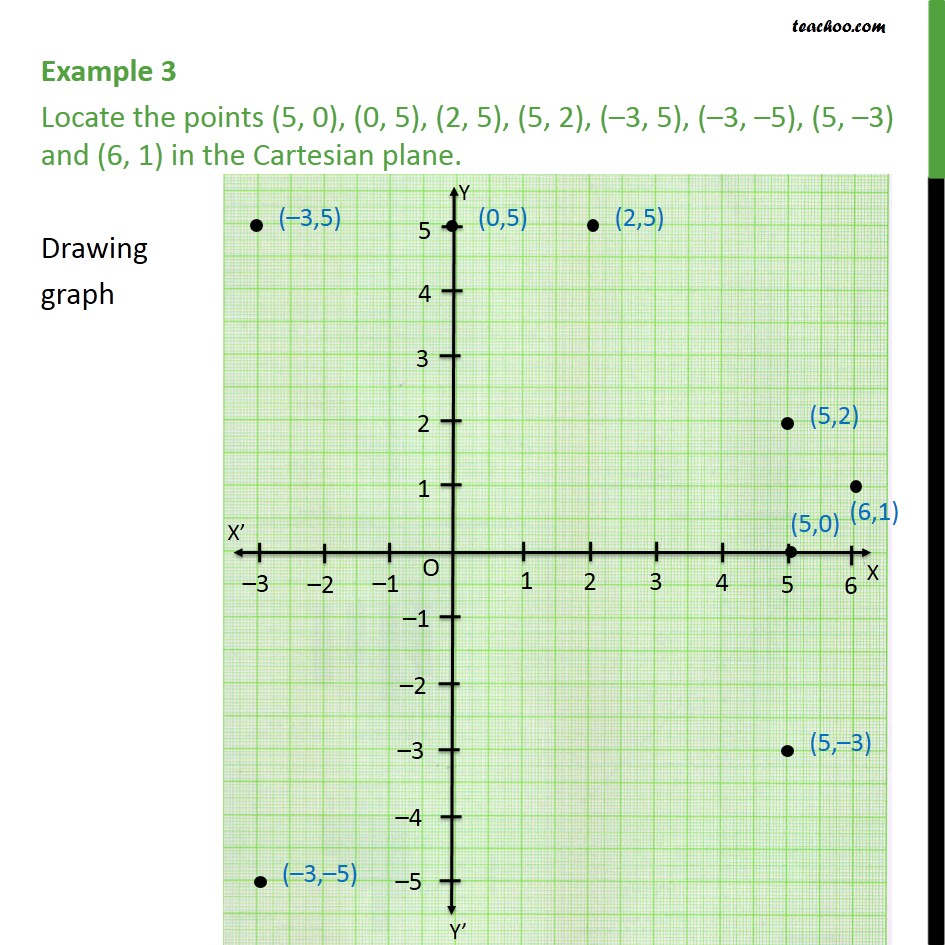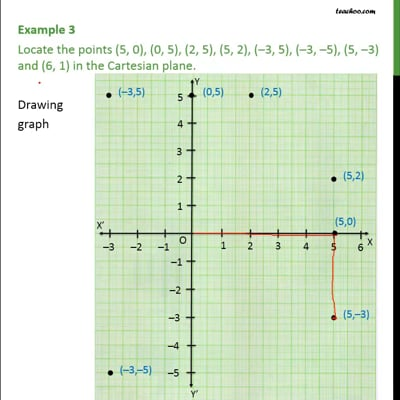Plotting points on the graph

Chapter 3 Class 9 Coordinate Geometry (Term 1)
Concept wiseThis video is only available for Teachoo black users

### Transcript

Example 3 Locate the points (5, 0), (0, 5), (2, 5), (5, 2), (–3, 5), (–3, –5), (5, –3) and (6, 1) in the Cartesian plane. We follow these steps to mark points 1. Drawing x and y axis 2. Now, in all points, maximum value of x is 6, and minimum is -3. So, we draw x-axis from -3 to 6 3. Also, in all points, maximum value of y is 5, and minimum is -3. So, we draw y-axis from -3 to 5 4. Let's mark points one by one 5. For (5, 0), we go to 5 in x-axis. Now, since y is 0. We mark that point. 6. Similarly, we can plot all points